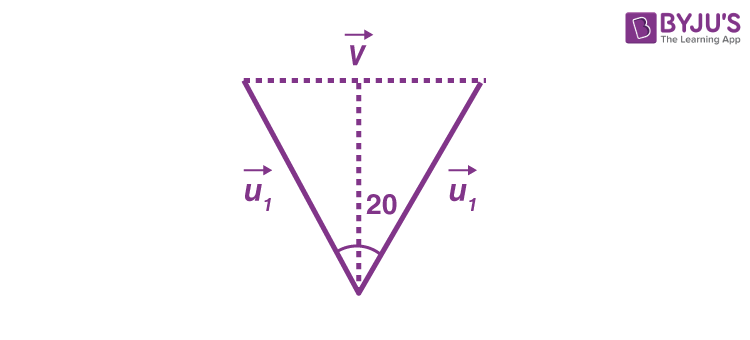# A cat runs along a straight line with a constant velocity of magnitude v. A dog chases the cat such that the velocity of the dog is always directed towards the cat. The speed of a dog is u and always constant. At the instant, both are separated by distance x and their velocities are mutually perpendicular, the magnitude of the acceleration of the dog is.

a) u2/x

b) uv/x

c) v2/x

d) (u2 + v2)/x

Solution:The velocity of cat = v

Speed of dog = u

Let the velocity of the dog be u1 and after some time its velocity is u2

By applying vector properties we get

vdθ = u2 -u——(1)

$$\begin{array}{l}\vec{a}=\frac{\vec{u_{2}}-\vec{u_{1}}}{dt}\end{array}$$

From equation (1)

$$\begin{array}{l}\vec{a}=\frac{vd\theta }{dt}\end{array}$$

When dθ is very small then dθ = Arc length/radius

dθ = udt/x

$$\begin{array}{l}\vec{a}=\frac{vudt }{xdt}\end{array}$$

a = uv/x(1)(0)